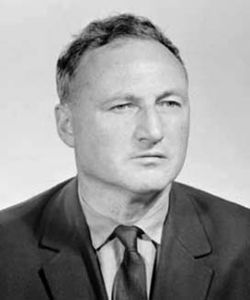### Born: 21 Jan 1915 in Belaya Tserkov, Ukraine Died: 30 June 1972 in Leningrad, USSR

Yuri Linnik's father was Vladimir Pavlovitch Linnik who was himself a famous scientist making major contributions to optics. Like his son, Vladimir was elected to the USSR Academy of Sciences.

After studying at secondary school Yuri Linnik worked as a laboratory assistant for a year in 1931. Then he entered Leningrad University (St Petersburg before 1914 and now St Petersburg again) to study mathematics and theoretical physics. He graduated in 1938, obtaining a doctorate there in 1940. In the same year he joined the Leningrad branch of the Steklov Institute for Mathematics.

From 1944 Linnik was professor of mathematics at Leningrad University in addition to his position in the Steklov Institute. He organised the chair of probability theory there and founded the Leningrad school of probability and mathematical statistics.

His main research topics were number theory, probability theory and mathematical statistics. He introduced ergodic methods into number theory in his first work. In a 1941 paper he introduced the large sieve method in number theory. In 1950 he introduced the ideas of probability into number theory and introduced the dispersion method in number theory.

Later Linnik made major contributions to probability with his work on limit theorems and was the first to use powerful techniques from analysis in mathematical statistics. He solved the Behrens-Fisher problem and many other difficult problems of mathematical statistics.

Linnik wrote several important texts including Characterisation Problems in Mathematical Statistics.

Linnik was President of the Moscow Mathematical Society for many years. He was honoured by the national mathematical society of Sweden and was awarded an honorary degree from Paris. He was elected to the USSR Academy of Sciences in 1964.

Article by: J.J. O'Connor and E.F. Robertson
Source:       MacTutor History of Mathematics archive